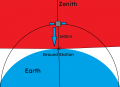# Is this calculated value correct? What does the max. distance mean?

#### Papabravo

Joined Feb 24, 2006
20,598
The max distance refers to how far a line-of sight signal at a given frequency is expected to propagate before the attenuation reaches a level where it cannot be demodulated to recover any useful information.

#### Solanum Asteridion

Joined Sep 5, 2023
36
The max distance refers to how far a line-of sight signal at a given frequency is expected to propagate before the attenuation reaches a level where it cannot be demodulated to recover any useful information.
Can you please explain through a diagram? I am not getting it.

#### Solanum Asteridion

Joined Sep 5, 2023
36
Also this app uses the Friis transmission equation. Is the calculated value correct?

#### Papabravo

Joined Feb 24, 2006
20,598
Can you please explain through a diagram? I am not getting it.
You have the diagram in your original post. It shows a satellite above a ground station at an altitude of 160 km which means it is in a low Earth orbit. The limits to line-of-sight transmission occur because of the curvature of the Earth. The other limitations have to do with transmitter power, receiver sensitivity, and signal attenuation along a path. The signal level of -176 dBm is understood to be the level where a signal drops below the noise floor rendering it undetectable. The max distance occurs along the path from the satellite to the horizon from an altitude of 160 km. The signal may or may not reach the horizon if it is low power or the receiver lacks sufficient sensitivity.

#### Papabravo

Joined Feb 24, 2006
20,598
Also this app uses the Friis transmission equation. Is the calculated value correct?
It is correct as far as the effects of the factors that it considers. Do you have reason to believe that it is wrong?

#### Solanum Asteridion

Joined Sep 5, 2023
36
You have the diagram in your original post. It shows a satellite above a ground station at an altitude of 160 km which means it is in a low Earth orbit. The limits to line-of-sight transmission occur because of the curvature of the Earth. The other limitations have to do with transmitter power, receiver sensitivity, and signal attenuation along a path. The signal level of -176 dBm is understood to be the level where a signal drops below the noise floor rendering it undetectable. The max distance occurs along the path from the satellite to the horizon from an altitude of 160 km. The signal may or may not reach the horizon if it is low power or the receiver lacks sufficient sensitivity.
So that means that if the satellite is in the LOS of the ground station, they will start communicating?

#### Solanum Asteridion

Joined Sep 5, 2023
36
It is correct as far as the effects of the factors that it considers. Do you have reason to believe that it is wrong?
It is a self-developed application that's why.

#### BobTPH

Joined Jun 5, 2013
8,076
It is a self-developed application that's why.
And you are asking us what the output means?

#### Papabravo

Joined Feb 24, 2006
20,598
It is a self-developed application that's why.
I am familiar with the concepts, but I am unable to verify your calculations. Maybe you could reveal the underlying equations you are using. That would help us to help you verify your results.

#### Solanum Asteridion

Joined Sep 5, 2023
36
sure thank you..

I first calculate the wavelength (lambda) using speed of light / frequency.

Then received power is calculated by Transmitted Power (in dBm) + Transmitter Gain (in dBi) + Receiver Gain (in dBi) - 20 * log10(Wavelength / (4 * π * Distance in meters))

Then it checks if received_power_dbm >= receiver_sensitivity_dbm, if so, the signal will be received by the ground station?

Finally, Maximum Distance (in kms) = ((Wavelength / (4 * π)) * 10^((Transmitted Power in dBm - Receiver Sensitivity in dBm + Transmitter Gain in dBi + Receiver Gain in dBi) / 20))/1000

#### Solanum Asteridion

Joined Sep 5, 2023
36
And you are asking us what the output means?
i am not sure if the application's formula is correct or not.

#### Papabravo

Joined Feb 24, 2006
20,598
sure thank you..

I first calculate the wavelength (lambda) using speed of light / frequency.

Then received power is calculated by Transmitted Power (in dBm) + Transmitter Gain (in dBi) + Receiver Gain (in dBi) - 20 * log10(Wavelength / (4 * π * Distance in meters))

Then it checks if received_power_dbm >= receiver_sensitivity_dbm, if so, the signal will be received by the ground station?

Finally, Maximum Distance (in kms) = ((Wavelength / (4 * π)) * 10^((Transmitted Power in dBm - Receiver Sensitivity in dBm + Transmitter Gain in dBi + Receiver Gain in dBi) / 20))/1000
Something does not make sense here. There should be no problem with transmission and reception between the ground station and the satellite overhead. I thought the problem was about transmission and reception from the satellite to a station at the periphery of the circular area of the sphere above the horizon from the satellite's point of view. So idk what you are talking about.